Definitions of Square Dance Calls and Concepts

\$B8~\$+\$\$9g\$C\$?%+%C%W%k\$+\$i!%(B

Swap The Top [C3A]:
Belles \$B\$O(B Extend (L-H) and Cast Off 3/4 \$B\$r\$7(B, Beaus \$B\$O(B Run once and a half \$B\$r\$7\$^\$9!%(B R-H Wave \$B\$G=*\$o\$j\$^\$9!%(B

Cross Swap The Top [C3B]:
Belles \$B\$O(B Cross Extend (R-H) and Cast Off 3/4 \$B\$r\$7(B, Beaus \$B\$O(B Run once and a half \$B\$r\$7\$^\$9!%(B R-H Two-Faced Line \$B\$G=*\$o\$j\$^\$9!%(B

Reverse Swap The Top [C3A]:
Beaus \$B\$O(B Extend (R-H) and Cast Off 3/4 \$B\$r\$7(B, Belles \$B\$O(B Run once and a half \$B\$r\$7\$^\$9!%(B L-H Wave \$B\$G=*\$o\$j\$^\$9!%(B

Reverse Cross Swap The Top [C3B]:
Beaus \$B\$O(B Cross Extend (L-H) and Cast Off 3/4 \$B\$r\$7(B, Belles \$B\$O(B Run once and a half \$B\$r\$7\$^\$9!%(B L-H Two-Faced Line \$B\$G=*\$o\$j\$^\$9!%(B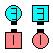\$B\$B;O\$a\$N(B formation: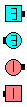Swap The Top\$B\$N8e(B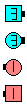Cross Swap The Top\$B\$N8e(B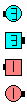Reverse Swap The Top\$B\$N8e(B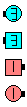Reverse Cross Swap The Top\$B\$N8e(B

\$B%@%s%9\$N%R%s%H(B: Run 1 and 1/2 \$B\$r\$9\$k?M\$O(B, \$B%3!<%k\$r;O\$a\$kA0\$K>/\$72<\$,\$C\$F\$+\$i9T\$&\$Y\$-\$G\$7\$g\$&!%(B

\$BJL\$NDj5A(B:

• Swap The Top: 1/2 Swap Around; Centers Cast Off 3/4 as Ends Roll twice .
• Reverse Swap The Top: 1/2 Reverse Swap Around; Centers Cast Off 3/4 as Ends Roll twice .
• Cross Swap The Top: 1/2 Swap Around; Centers Slither; Centers Cast Off 3/4 as Ends Roll twice .
• Reverse Cross Swap The Top: 1/2 Reverse Swap Around; Centers Slither; Centers Cast Off 3/4 as Ends Roll twice .

\$B\$?\$V\$s(B Swap The Top \$B\$O(B Swap Your Neighbor \$B\$HL?L>\$5\$l\$k\$Y\$-\$G\$7\$?(B.